Ex 5.1

Chapter 5 Class 12 Continuity and Differentiability
Serial order wise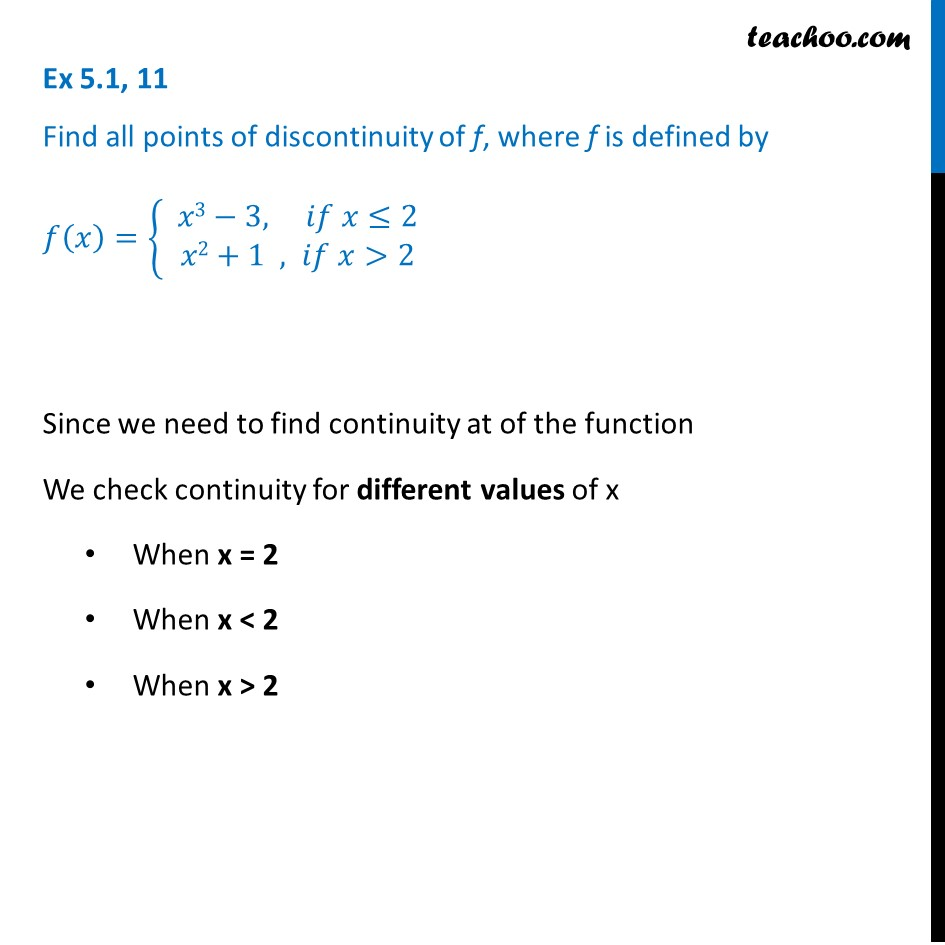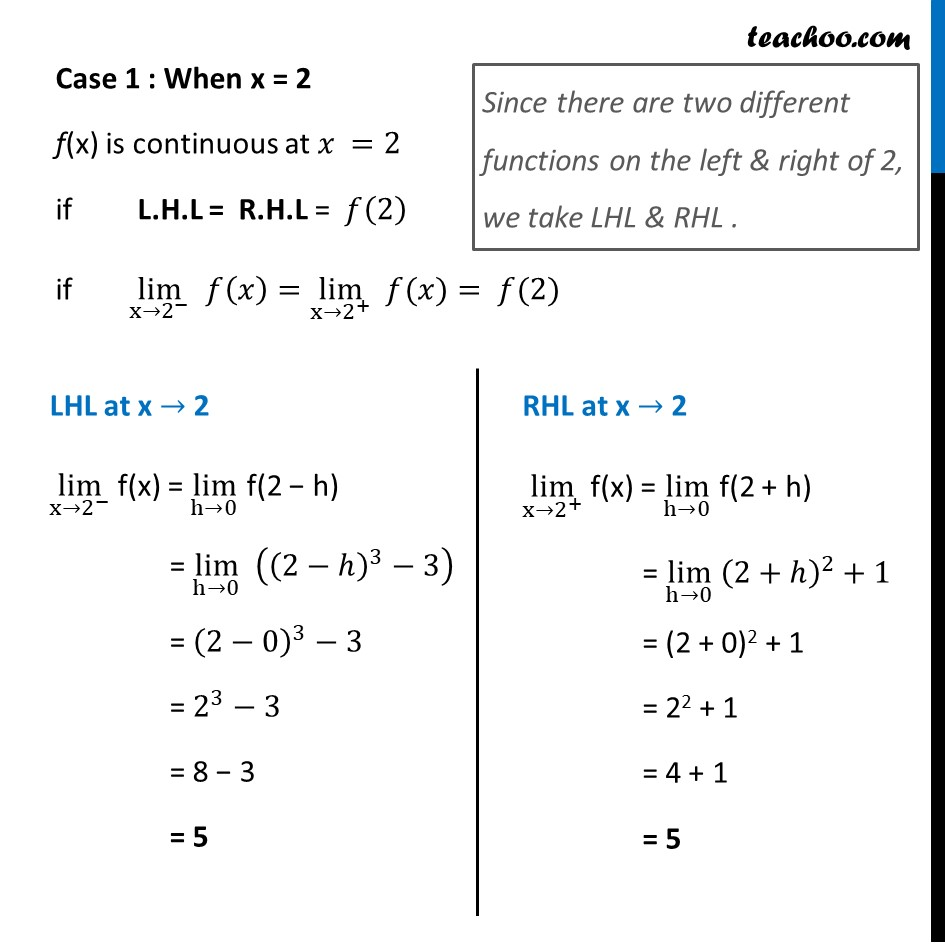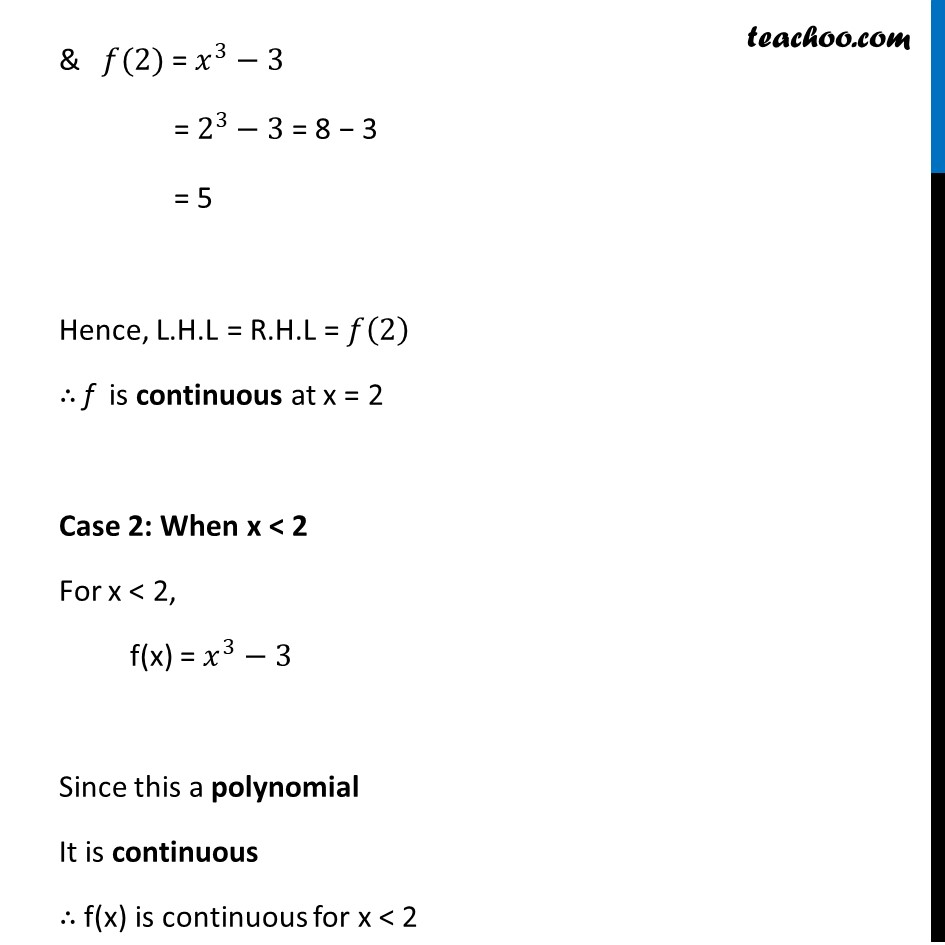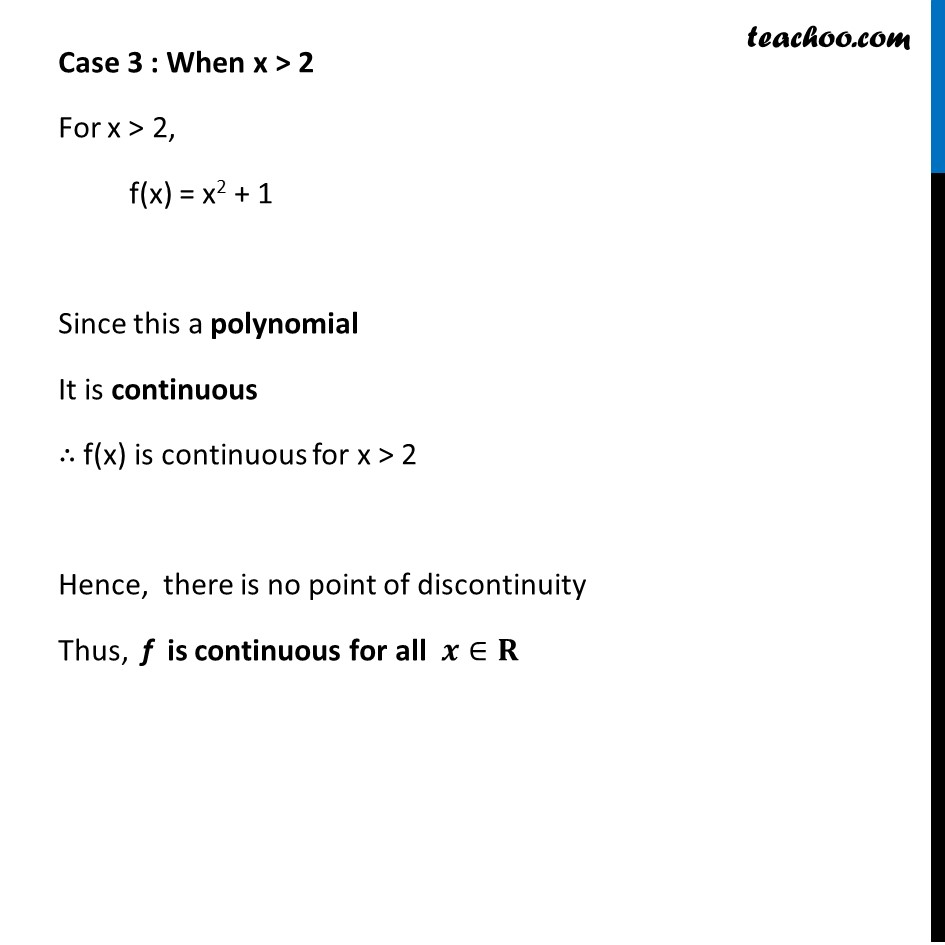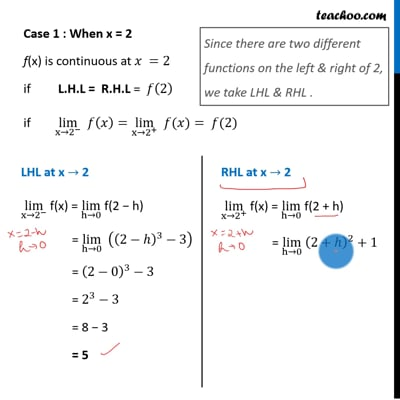This video is only available for Teachoo black users

Introducing your new favourite teacher - Teachoo Black, at only ₹83 per month

### Transcript

Ex 5.1, 11 Find all points of discontinuity of f, where f is defined by 𝑓(𝑥)={ █(𝑥3−3, 𝑖𝑓 𝑥≤2@&𝑥2+1 , 𝑖𝑓 𝑥>2)┤ Since we need to find continuity at of the function We check continuity for different values of x When x = 2 When x < 2 When x > 2 Case 1 : When x = 2 f(x) is continuous at 𝑥 =2 if L.H.L = R.H.L = 𝑓(2) if lim┬(x→2^− ) 𝑓(𝑥)=lim┬(x→2^+ ) " " 𝑓(𝑥)= 𝑓(2) Since there are two different functions on the left & right of 2, we take LHL & RHL . LHL at x → 2 lim┬(x→2^− ) f(x) = lim┬(h→0) f(2 − h) = lim┬(h→0) ((2−ℎ)^3−3) = (2−0)^3−3 = 2^3−3 = 8 − 3 = 5 RHL at x → 2 lim┬(x→2^+ ) f(x) = lim┬(h→0) f(2 + h) = lim┬(h→0) (2+ℎ)^2+1 = (2 + 0)2 + 1 = 22 + 1 = 4 + 1 = 5 & 𝑓(2) = 𝑥^3−3 = 2^3−3 = 8 − 3 = 5 Hence, L.H.L = R.H.L = 𝑓(2) ∴ f is continuous at x = 2 Case 2: When x < 2 For x < 2, f(x) = 𝑥^3−3 Since this a polynomial It is continuous ∴ f(x) is continuous for x < 2 Case 3 : When x > 2 For x > 2, f(x) = x2 + 1 Since this a polynomial It is continuous ∴ f(x) is continuous for x > 2 Hence, there is no point of discontinuity Thus, f is continuous for all 𝒙∈𝐑